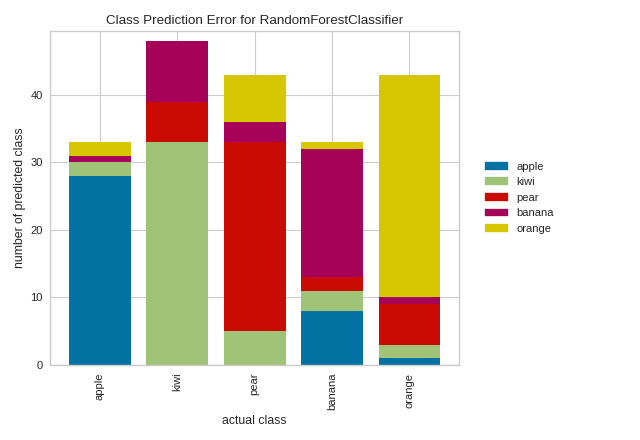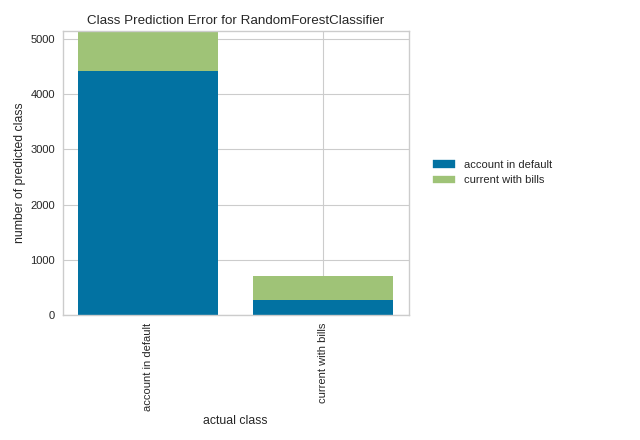# Class Prediction Error¶

The Yellowbrick ClassPredictionError plot is a twist on other and sometimes more familiar classification model diagnostic tools like the Confusion Matrix and Classification Report. Like the Classification Report, this plot shows the support (number of training samples) for each class in the fitted classification model as a stacked bar chart. Each bar is segmented to show the proportion of predictions (including false negatives and false positives, like a Confusion Matrix) for each class. You can use a ClassPredictionError to visualize which classes your classifier is having a particularly difficult time with, and more importantly, what incorrect answers it is giving on a per-class basis. This can often enable you to better understand strengths and weaknesses of different models and particular challenges unique to your dataset.

The class prediction error chart provides a way to quickly understand how good your classifier is at predicting the right classes.

from sklearn.datasets import make_classification
from sklearn.model_selection import train_test_split
from sklearn.ensemble import RandomForestClassifier
from yellowbrick.classifier import ClassPredictionError

# Create classification dataset
X, y = make_classification(
n_samples=1000, n_classes=5, n_informative=3, n_clusters_per_class=1,
random_state=36,
)

classes = ["apple", "kiwi", "pear", "banana", "orange"]

# Perform 80/20 training/test split
X_train, X_test, y_train, y_test = train_test_split(X, y, test_size=0.20,
random_state=42)
# Instantiate the classification model and visualizer
visualizer = ClassPredictionError(
RandomForestClassifier(random_state=42, n_estimators=10), classes=classes
)

# Fit the training data to the visualizer
visualizer.fit(X_train, y_train)

# Evaluate the model on the test data
visualizer.score(X_test, y_test)

# Draw visualization
visualizer.poof()In the above example, while the RandomForestClassifier appears to be fairly good at correctly predicting apples based on the features of the fruit, it often incorrectly labels pears as kiwis and mistakes kiwis for bananas.

By contrast, in the following example, the RandomForestClassifier does a great job at correctly predicting accounts in default, but it is a bit of a coin toss in predicting account holders who stayed current on bills.

from sklearn.model_selection import train_test_split
from sklearn.ensemble import RandomForestClassifier
from yellowbrick.classifier import ClassPredictionError
from yellowbrick.datasets import load_credit

X, y = load_credit()

classes = ['account in default', 'current with bills']

# Perform 80/20 training/test split
X_train, X_test, y_train, y_test = train_test_split(X, y, test_size=0.20,
random_state=42)

# Instantiate the classification model and visualizer
visualizer = ClassPredictionError(
RandomForestClassifier(n_estimators=10), classes=classes
)

# Fit the training data to the visualizer
visualizer.fit(X_train, y_train)

# Evaluate the model on the test data
visualizer.score(X_test, y_test)

# Draw visualization
visualizer.poof()## API Reference¶

Shows the balance of classes and their associated predictions.

class yellowbrick.classifier.class_prediction_error.ClassPredictionError(model, ax=None, classes=None, is_fitted='auto', **kwargs)[source]

Bases: yellowbrick.classifier.base.ClassificationScoreVisualizer

Class Prediction Error chart that shows the support for each class in the fitted classification model displayed as a stacked bar. Each bar is segmented to show the distribution of predicted classes for each class. It is initialized with a fitted model and generates a class prediction error chart on draw.

Parameters
model: estimator

Scikit-Learn estimator object. Should be an instance of a classifier, else __init__() will raise an exception. If the internal model is not fitted, it is fit when the visualizer is fitted, unless otherwise specified by is_fitted.

ax: axes, default: None

the axis to plot the figure on.

classes: list, default: None

A list of class names for the legend. If classes is None and a y value is passed to fit then the classes are selected from the target vector.

is_fittedbool or str, default=”auto”

Specify if the wrapped estimator is already fitted. If False, the estimator will be fit when the visualizer is fit, otherwise, the estimator will not be modified. If “auto” (default), a helper method will check if the estimator is fitted before fitting it again.

kwargs: dict

Keyword arguments passed to the super class. Here, used to colorize the bars in the histogram.

Notes

These parameters can be influenced later on in the visualization process, but can and should be set as early as possible.

Attributes
score_float

Global accuracy score

predictions_ndarray

An ndarray of predictions whose rows are the true classes and whose columns are the predicted classes

draw(self)[source]

Renders the class prediction error across the axis.

Returns
axMatplotlib Axes

The axes on which the figure is plotted

finalize(self, **kwargs)[source]

Finalize executes any subclass-specific axes finalization steps. The user calls poof and poof calls finalize.

score(self, X, y, **kwargs)[source]

Generates a 2D array where each row is the count of the predicted classes and each column is the true class

Parameters
Xndarray or DataFrame of shape n x m

A matrix of n instances with m features

yndarray or Series of length n

An array or series of target or class values

Returns
score_float

Global accuracy score